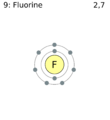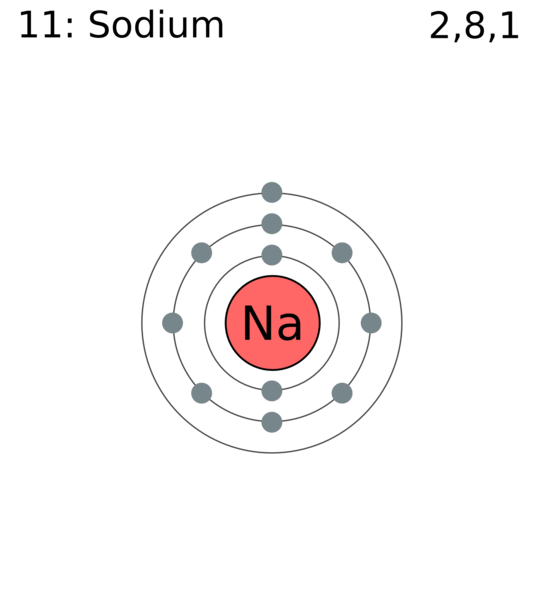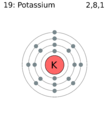# Electrons and Valence for Simple Biologically Active Ions

$$\newcommand{\vecs}{\overset { \rightharpoonup} {\mathbf{#1}} }$$ $$\newcommand{\vecd}{\overset{-\!-\!\rightharpoonup}{\vphantom{a}\smash {#1}}}$$$$\newcommand{\id}{\mathrm{id}}$$ $$\newcommand{\Span}{\mathrm{span}}$$ $$\newcommand{\kernel}{\mathrm{null}\,}$$ $$\newcommand{\range}{\mathrm{range}\,}$$ $$\newcommand{\RealPart}{\mathrm{Re}}$$ $$\newcommand{\ImaginaryPart}{\mathrm{Im}}$$ $$\newcommand{\Argument}{\mathrm{Arg}}$$ $$\newcommand{\norm}{\| #1 \|}$$ $$\newcommand{\inner}{\langle #1, #2 \rangle}$$ $$\newcommand{\Span}{\mathrm{span}}$$ $$\newcommand{\id}{\mathrm{id}}$$ $$\newcommand{\Span}{\mathrm{span}}$$ $$\newcommand{\kernel}{\mathrm{null}\,}$$ $$\newcommand{\range}{\mathrm{range}\,}$$ $$\newcommand{\RealPart}{\mathrm{Re}}$$ $$\newcommand{\ImaginaryPart}{\mathrm{Im}}$$ $$\newcommand{\Argument}{\mathrm{Arg}}$$ $$\newcommand{\norm}{\| #1 \|}$$ $$\newcommand{\inner}{\langle #1, #2 \rangle}$$ $$\newcommand{\Span}{\mathrm{span}}$$$$\newcommand{\AA}{\unicode[.8,0]{x212B}}$$

#### Electrons and Valence in Biology

We noted that it is necessary to understand the electronic structure of atoms to explain light, color, photochemistry (photosynthesis and vision), and size and shape of molecules.

But a model for electronic structure is also necessary to explain properties of atoms and chemical bonding. We also have seen that [Chemical and Biological Properties of Some Groups of Elements |alkali metal- and halogen groups] each have properties in common. We should be able to explain why alkali metals form 1+ ions, and appear to be suitable for nerve conduction, in terms of electronic configurations. The same goes for halogens forming 1- ions.

On a chemical level, an important clue to the unraveling of is the existence of noble gases, which are almost completely unable to form chemical compounds. This lack of reactivity suggests that the atoms of these elements have structures which do not permit interaction with the structures of other atoms. A second clue is the close correspondence between the valence of an element and the extent to which its atomic number differs from that of the nearest noble gas. Elements which have a valence of 1, for instance, have atomic numbers one more or one less than that of a noble gas. Thus the atoms of the alkali metals Li, Na, K, Rb, and Cs all contain one electron more than the corresponding noble gases He, Ne, Ar, Kr, and Xe, while atoms of hydrogen H and the halogens F, Cl, Br, and I all contain one electron less. Note in the crude sketches below that Neon has filled first (2 e) and second (8 e) shells. F has one less in the second shell, so it tend to react chemical by gaining an electron forming F-, while Na has 1 more (in the third shell), so it tends to lose the electron to form the stable Ne configuration, as K+.Figure $$\PageIndex{1}$$ Fluorine Shell ModelFigure $$\PageIndex{2}$$ Neon Shell ModelFigure $$\PageIndex{3}$$ Sodium Shell Model

The elements just below these in the periodic chart have similar outer electron configurations, so react similarly, as predicted by the periodic law.Figure $$\PageIndex{4}$$ Chlorine Shell ModelFigure $$\PageIndex{5}$$ Argon Shell ModelFigure $$\PageIndex{6}$$ Potassium Shell Model

Similar remarks apply to a valence of 2. The alkaline-earth metal atoms Be, Mg, Ca, Sr, and Ba all contain two electrons more than a noble-gas atom, while the elements O, S, Se, and Te all contain two electrons less. So Mg forms the Mg2+ ion, and O forms the O2- ion. Exactly the same pattern of behavior also extends to elements with a valence of 3 or 4.Figure $$\PageIndex{7}$$ Oxygen Shell ModelFigure $$\PageIndex{8}$$ Neon Shell ModelFigure $$\PageIndex{9}$$ Magnesium Shell Model

As early as 1902, Lewis began to suggest (in his lectures to general chemistry students, no less) that the behavior just described could be explained by assuming that the electrons in atoms were arranged in shells, all electrons in the same shell being approximately the same distance from the nucleus. He develope pictures like the ones above, with his figures here:Figure $$\PageIndex{10}$$The shell structure of atoms of He, Cl, and K, as suggested by Lewis.

In the helium atom the two electrons occupy only one shell, in the chlorine atom the 17 electrons are arranged in three shells, and in the potassium atom the 19 electrons occupy four shells. Lewis suggested that each shell can only accommodate so many electrons. Once this number has been reached, the shell must be regarded as filled, and any extra electrons are accommodated in the next shell, somewhat farther from the nucleus. Once a shell is filled, moreover, it is assumed to have a particularly stable structure which prevents the electrons in the shell from any involvement with other atoms. Thus it is only the electrons in the outermost incompletely filled shell (called valence electrons) that have any chemical importance. Furthermore, if the outermost shell is filled, then the resulting atom will have little or no tendency to react with other atoms and form compounds with them. Since this is exactly the behavior exhibited by the noble gases, Lewis concluded that the characteristic feature of an atom of a noble gas is a filled outer shell of electrons.

Assuming that the noble gases all contain an outermost filled shell, it is now quite simple to work out how many electrons can be accommodated in each shell. Since the first noble gas helium has two electrons, we know that only two electrons are needed to fill the first shell. A further eight electrons brings us to the next noble gas neon (Z = 10). Accordingly we deduce that the second shell can accommodate a maximum of eight electrons. A similar argument leads to the conclusion that the third shell also requires eight electrons to fill it and that an atom of argon has two electrons in the first shell, eight in the second, and eight in the third, a total of 18 electrons.Vitz 13:13, 2 October 2009 (UTC)

From ChemPRIME: 5.1: Electrons and Valence

## References

1. commons.wikimedia.org/wiki/Category:Electron_shell_diagrams
2. commons.wikimedia.org/wiki/Category:Electron_shell_diagrams
3. commons.wikimedia.org/wiki/Category:Electron_shell_diagrams
4. commons.wikimedia.org/wiki/Category:Electron_shell_diagrams
5. commons.wikimedia.org/wiki/Category:Electron_shell_diagrams
6. commons.wikimedia.org/wiki/Category:Electron_shell_diagrams
7. commons.wikimedia.org/wiki/Category:Electron_shell_diagrams
8. http://commons.wikimedia.org/wiki/Category:Electron_shell_diagrams
9. http://commons.wikimedia.org/wiki/Category:Electron_shell_diagrams